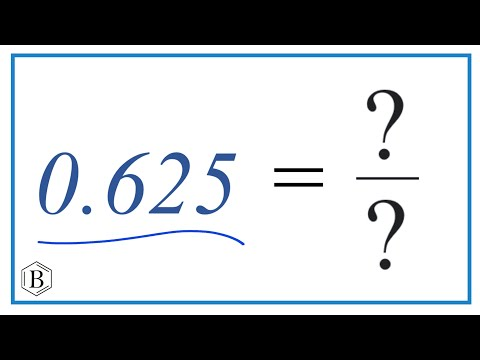Home » What is 0.625 as a Fraction? – Decimal to Fraction Conversion

# What is 0.625 as a Fraction? – Decimal to Fraction Conversion

What is 0.625 as a Fraction?

• A. 5/8
• B. 7/20
• C. 1/2
• D. 3/5
• E. 129/100

## Solution:

0.625 can be expressed as the fraction 5/8.

Here’s a quick explanation:

Since 0.625 is a decimal in the thousandth place, it can be expressed as a fraction over 1000 times. So, 625/1000 is where we start.

The greatest common divisor (GCD) of 625 and 1000, which is 125, can simplify this fraction. You get 5/8 if you divide the numerator (625) and denominator (1000) by 125.

So therefore, 0.625 as a fraction is equal to 5/8.

## Understanding the Decimal Place

Understanding the decimal place value is the first step in converting a decimal to a fraction. In this case, the decimal value is 0.625. The ‘625’ in this case refers to the thousandths. This is due to the fact that in the decimal number system, the first digit to the right of the decimal point denotes tenths, the second, hundredths, and the third, thousandths. As a result, we can first represent the decimal 0.625 as the fraction 625/1000.

## Simplifying the Fraction

The next step is to make this fraction as easy as possible. When the greatest common divisor (GCD) of the numerator and the denominator is 1, the fraction is in its simplest form. This means that the only thing the numerator and denominator have in common is 1. In the case of 625/1000, the largest number that both 625 and 1000 can be divided by is 125.

## 0.625 as a Fraction in Simplest Form

To simplify the fraction 625/1000, the numerator (625) and denominator (1000) are divided by their GCD, 125. When we do that, we get 5/8 (because 625 ÷ 125 = 5 and 1000 ÷ 125 = 8).

Thus, the simplest fractional representation of the decimal 0.625 is 5/8. This method can be used to convert any decimal to its fractional equivalent. To convert decimals to fractions, one must understand decimal place values, express the decimal as a fraction, and then, if necessary, simplify that fraction.